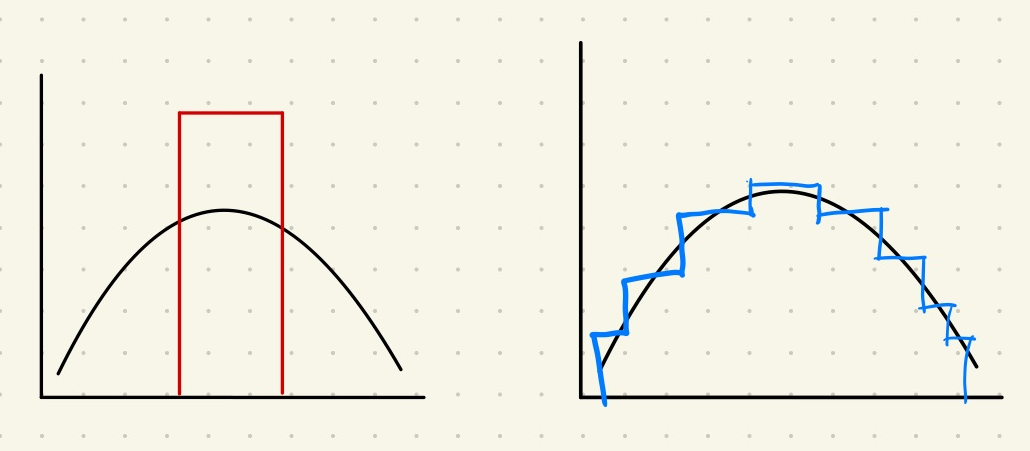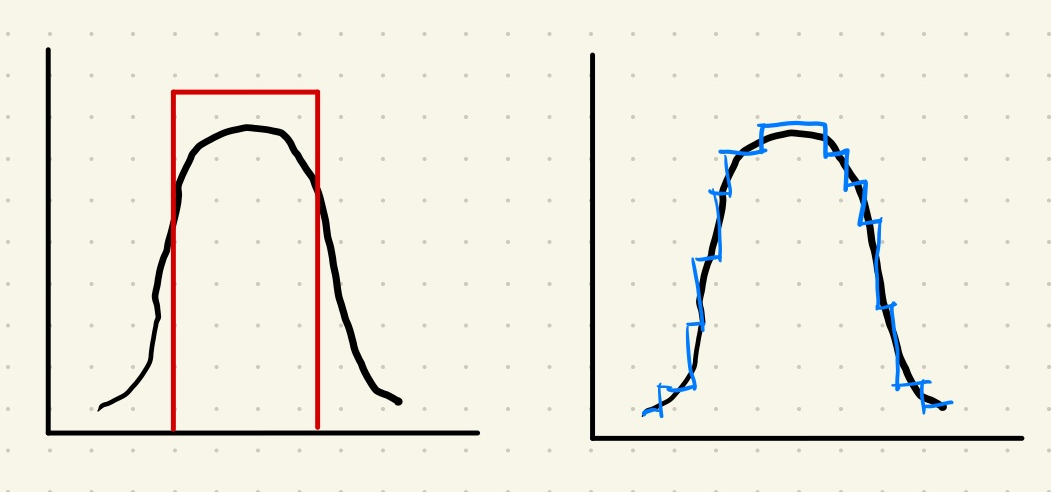Suppose we have a generating process that generates some numbers based on a distribution. Based on a data sample, we could reconstruct some sort of theoretical models to represent the actual generating process.Which is a Good Model? (1)The black curve represent the generating process. The red rectangle is a very simple model that captures some major samples. The blue step-wise model is capturing more sample data but with more parameters.

In the above example, the red model on the left is not that good in most cases while the blue model seems to be better. In reality, the choice depends on the usage of the model. But we can already tell that the balance is between how well the model describes the data and how complicated the model is.

To make it even more conflicting, the following illustration shows another generating process and two corresponding models.Which is a Good Model? (2)The black curve represent the generating process. The red rectangle is a very simple model that captures some major samples. The blue step-wise model is capturing more sample data but with more parameters.

In this case, we might agree that the red simple model is probably good enough for many situations. While the blue model is captures more features of the data, we have to deal with more parameters.

## What is a Good Model?

Presumably, a good model should be

• plausibility (we do not like models that explain suicide rates primarily based on the coverage of Internet Explorer),
• balance of parsimony and goodness-of-fit (we can not use models that perform badly but a good-performing model with ten-thousand parameters is not exactly a good one either most of the time),
• coherence of the underlying assumptions,
• easy to understand when it breaks down,
• consistency with known results,
• especially with the simple and basic phenomena,
• ability to explain rather than describe data,
• extent to which model predictions can be falsified through experiments.

Parsimony

The parsimony concept is a natural consequence of Occam’s razor: We choose the simple model for more explanatory power.

For example, the instance theory by Logan is a good model to explain the lexical decision task. It is not a perfect model, but it bears parsimony.

## How to choose a model?

To choose a good model, we need a framework to compare two models. The comparison shall at least address the goodness-of-fit and parsimony.

Many methods have been proposed to deal with the balance between parsimony and goodness-of-fit, e.g.,

• Information criteria (IC) such as and ,
• Minimum description length ( ),
• Bayes factors.

Here we demonstrate how IC can tell us which model is better. We calculate the IC of all the models at hand and specify the delta

$$\Delta _i = \mathrm{IC}_i - \operatorname{min} \mathrm{IC}.$$

Then we specify the weights of models

$$w_i = \frac{ \exp\{-\Delta_i/2\} }{ \sum_{m=1}^M \exp\{-\Delta_m/2\} }.$$

The model with larger weight $w_i$ is a better model.

Akaike weight and Schwarz weight

If we use AIC as the IC in the formula, this weight $w_i$ is called Akaike weight; If we use BIC, the weight $w_i$ is called Schwarz weight.

There are other criteria too. For example, we can use the minimum description lengthor the Bayes factors.

Planted: by ;

L Ma (2020). 'Model Selection', Datumorphism, 11 April. Available at: https://datumorphism.leima.is/wiki/model-selection/model-selection/.